### Model and Item Fit

The IRT procedure includes five model fit statistics: log likelihood, Akaike’s information criterion (AIC), Bayesian information criterion (BIC), and likelihood ratio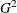.

The following equation computes the likelihood ratio,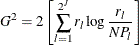where N is the number of subjects,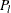is the estimated probability of observing response pattern l, and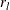is the number of subjects who have response pattern l. When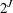, the number of possible response patterns, is much greater than N, the frequency table is sparse; this invalidates the use of chi-square distribution as the asymptotic distribution for these two statistics, and as a result they should not be used to evaluate overall model fit.

For item fit, PROC IRT computes the likelihood ratioand Pearson’s chi-square. The Pearson’s chi-square statistic, proposed by Yen (1981), has the form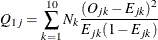The likelihood ratio, proposed by McKinley and Mills (1985), uses the following equation: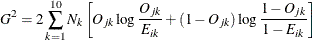To calculate these two statistics, first order all the subjects based on their estimated factor scores, and then partition them into 10 intervals such that the numbers of subjects in each interval are approximately equal.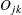and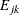are the observed and expected proportions of subjects in interval k who have a correct response on item j. The expected proportionsare computed as the mean predicted probability of a correct response in interval k.